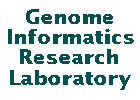PRACTICAL
 UPF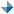IMIMGRIBHOMECOURSESSEQUENCE ANALYSISSEARCH BY SIGNALProfiles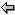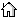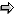An Introduction to Profiles

#### by Roderic Guigo, IMIM and UB, Barcelona

DISCLAIMER: This document is only an exercise on javascript. There are bugs. It has been only tested on Netscape clients---version 3 and higher--- running on Silicon Graphics.

A Profile or Position Weigth Matrix (the two terms are used synonymously here) is a motif descriptor. It attempts to capture the intrinsic variability characteristic of sequence patterns. A Profile it is usually derived from a set of aligned sequences functionally related. For instance, below we have the sequence of ten vertebrate donor sites, aligned at the boundary exon/intron.
sequence   1:
sequence   2:
sequence   3:
sequence   4:
sequence   5:
sequence   6:
sequence   7:
sequence   8:
sequence   9:
sequence 10:

Position Weight Matrix

We derive a Profile from above set of sequences by tabulating the frequency with which each nucleotide is observed at each position. Click on "Calculate Matrix" above to obtain such observed frequencies.
Formally, from a set S of n aligned sequences of length l, s1, ... , sn, where sk = sk1, ... , skl (the skj being one of {A, C, G, T} in the case of DNA sequences) a Position Weigth Matrix, M4xl is derived as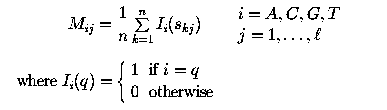Each coefficient in this matrix indicates the number of times that a given nucleotide has been observed at a given position. For instance, the nucleotide "A" has been observed in three of the aligned sequences in position 1, and so is indicated in the matrix. Note also, that in this case two positions are absolutely conserved, postions 4 and 5 corresponding to the mandatory dinucleotide GT at the begining of the intron. Of course, different sets of aligned sequences result in different profiles. You can play with the input sequences, and see how the profile changes when the aligned sequences change.

More often than the absolute frequencies, the relative frequencies are tabulated in a profile. In such a case, the coefficients of the matrix can be interpreted as probabilities of a given nucleotide occurring at a given position in a functional site. Then, given a sequence of length l, the product of the coefficients from such a matrix correspoding to each nucleotide in each position of the sequence is the probability of finding such a sequence in a true functional site. For instance, the probability of finding the sequence CAGGTTGGA in the functional site described by the matrix above (assuming that you have not changed the original input sequences) is 0.20x0.59x0.69x1x1x0.10x0.10x0.5x0.10. While the probability of finding such a sequence in a random site is the product of the "a priori" probabilities of the corresponding nucleotides. For instance, if we assume that all nucleotides are equally probable, such a probability is simply 0.259. The ratio between the probability of a sequence in a functional site and the probability of a sequence in a random site is a likelihood ratio, and its logarithm a log likelihood ratio. Such a ratio is equal to zero if a sequence has the same probability to appear in a functional site than in a random site, is greater than zero if the sequence is more likely to be found in a functional site than in a random site, and smaller than zero the other way around.

Often, thus, the coefficients in a Position Weigth Matrix are directly computed as log-likelyhood values according with the following transformation log(Mij/pi), where Mij is the probability of nucleotide i at position j in the Matrix M, and pi is the background probability of nucleotide i. The background probability of nucleotide can be assumed to be an "a priory" probability, the frequency of the nucleotide in the whole sequences use to derive the matrix, or the frequency in the aligned region from where the matrix is actually derived. Then, given a sequence of length l above log-likelihood ratio can be computed by summing the coefficients of the log-likelihood matrix corresponding to each nucleotide in each position on the sequence.

Below you can see how the absolute frequencies matrix that you have originally derived is transformed to a relative frequencies matrix, or a log likelihood matrix (assuming all nucleotides equiprobable).

Once a Profile has been derived from a set of functionally related sites, the Profile can be used to scan a query sequence for the presence of potential sites. Usually you run a window the length of the matrix along the sequence, and sum the coefficients from the matrix corresponding to each nucleotide in each position on the window sequence. Formally, the score of a matrix M for a site s of length l (s = s1, ... , sl, and sk being one of {A, C, G, T}) is computed as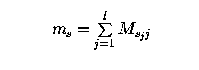You can use any form of above matrix to search for occurrences of the motif in a given sequence, but if you use the log-likelihood matrix, the scores that you will obtain are log-likelihood ratios. You can use the sequence below or your own sequence, and see how the scores along each position in the sequence are caculated.

use the buttons to scan the Profile along the sequence
 1 2 3 4 5 6 7 8 9 A C G T
 Score
As a result of scaning the sequence with the matrix, you obtain an score at each position. Click on 'ScanSequence' for the whole list of scores along the sequence.

Sometimes you may want to plot the scores graphically
But usually you are only interested in the scores over a given threshold. Often, you set such a threshold at the minimum value scored by the sequences from which the profile has been derived. For instance, the minimum value scored by the original sequences from which above profile has been derived is

Using this threshold, you obtain a reduced list of matches,

and a clearer plot
Of course, you can play with the threshold and increase or decrease the number of potential matches, incresing and decreasing accordingly sensitivity and specificity (Change the value of the threshold and click on "ScanSequence" afterwords.)

 Disclaimer